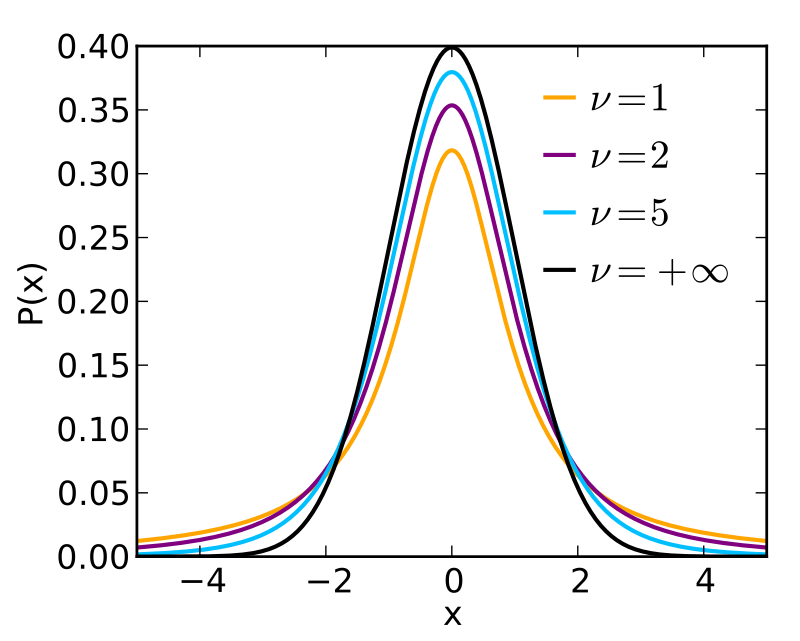# Further hypothesis testing, confidence intervals and regions

10/07/2020

## Instructions:

Use the left and right arrow keys to navigate the presentation forward and backward respectively. You can also use the arrows at the bottom right of the screen to navigate with a mouse.

FAIR USE ACT DISCLAIMER:
This site is for educational purposes only. This website may contain copyrighted material, the use of which has not been specifically authorized by the copyright holders. The material is made available on this website as a way to advance teaching, and copyright-protected materials are used to the extent necessary to make this class function in a distance learning environment. The Fair Use Copyright Disclaimer is under section 107 of the Copyright Act of 1976, allowance is made for “fair use” for purposes such as criticism, comment, news reporting, teaching, scholarship, education and research.

## Outline

• The following topics will be covered in this lecture:
• Testing a single predictor
• The t-test
• Testing a subspace
• Confidence intervals
• Confidence regions

## Testing one predictor

• As a general method, we can always use the F-statistic for nested models.

• Specifically, whenever one model is given by a subspace of another:

• $$\boldsymbol{\omega}$$ consists of models over variables $$x_1, \cdots, x_{q-1}$$ and corresponds to $$q$$ parameters (including the intercept);
• $$\boldsymbol{\Omega}$$ consists of models over variables $$x_1, \cdots , x_{p-1}$$ and corresponds to $$p$$ parameters (including the itercept);
• such that $$q \text{<} p$$.
• Concretely, the null hypothesis must be $$H_0 : \boldsymbol{\beta}_i = \boldsymbol{0}$$ for each $$i=q,\cdots, p-1$$.

• The alternative hypothesis is that the larger model holds,

$H_1: \boldsymbol{\beta} \neq 0$

• We compute the F statistic as, \begin{align} F &\triangleq \frac{ \left( RSS_\boldsymbol{\omega} - RSS_\boldsymbol{\Omega}\right)/ (p-1)}{RSS_\boldsymbol{\Omega}/(n-p)} . \end{align}

### Testing one predictor – continued

• Suppose there is one particular variable that we want to determine the significance of for our model.

• Specifically, suppose we have a model,

\begin{align} \mathbf{Y} = \mathbf{X} \boldsymbol{\beta} + \boldsymbol{\epsilon}, \end{align} with respect to some choice of variables $$\mathbf{X}$$.

• Our alternative hypothesis in this case is,

\begin{align} H_1: \boldsymbol{\beta} \neq \boldsymbol{0}. \end{align}

• Q: if we want to determine if $$\boldsymbol{\beta}_i$$ specifically gives an appreciable difference in this model, what is our null hypothesis?

• A: our null hypothesis takes the form,

\begin{align} H_0: \boldsymbol{\beta}_i = 0 \end{align}

### Testing one predictor – continued

• We will examine this on the gala data once again.

• We define the model lmods without area as an explanatory variable for the null hypothesis. Then we compute the ANOVA table with the bigger model that contains area

require("faraway")
lmod <- lm(Species ~ Area + Elevation + Nearest + Scruz + Adjacent, gala)
lmods <- lm(Species ~ Elevation + Nearest + Scruz + Adjacent, gala)
anova(lmods, lmod)

Analysis of Variance Table

Model 1: Species ~ Elevation + Nearest + Scruz + Adjacent
Model 2: Species ~ Area + Elevation + Nearest + Scruz + Adjacent
Res.Df   RSS Df Sum of Sq      F Pr(>F)
1     25 93469
2     24 89231  1    4237.7 1.1398 0.2963

• The result of the $$F$$ test is to say, “With probability 29.63%, we will find a value drawn from the F distribution with this value or greater”.

• Q: do we reject or fail to reject the null hypothesis at $$5\%$$ significance here?

• A: Here we fail to reject the null hypothesis because it is reasonable that the difference between the large model and the small model could be due to random variation.

• Note: there may be some statistical relationship, but we haven't detected one that wouldn't be surpising if it was just noise.

### Student t-distributionCourtesy of Skbkekas CC BY-SA 3.0

• The significance of a single variable can also be found with respect to the student t-test.
• Generally, suppose that we have $$n$$ independent random variables drawn from a Gaussian distribution $$\left\{Y_i\right\}_{i=1}^n$$, with unknown true mean $$\mu_Y$$ and standard deviation $$\sigma$$.
• As usual, our sample-based estimate of the mean is given by, \begin{align} \overline{Y} = \frac{1}{n}\sum_{i=1}^n Y_i ; \end{align}
• and our unbiased, sample-based estimate of the variance is given as, \begin{align} S^2 = \frac{1}{n-1} \sum_{i=1}^n \left(Y_i - \overline{Y}\right)^2. \end{align}
• It is a powerful and non-trivial result that, \begin{align} \frac{\overline{Y} - \mu_Y}{S/ \sqrt{n}}, \end{align} is distributed according to a student t-distribution, in $$n-1$$ degrees of freedom.